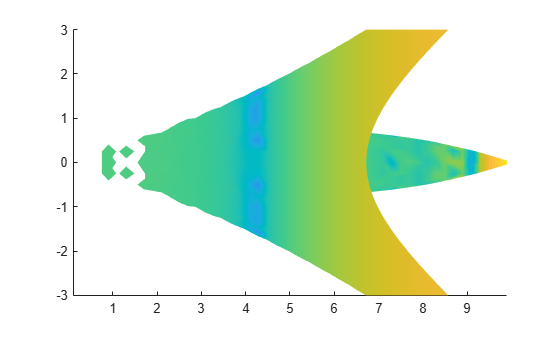# volumebounds

Coordinate and color limits for volume data

## Syntax

```lims = volumebounds(X,Y,Z,V) lims = volumebounds(X,Y,Z,U,V,W) lims = volumebounds(V) lims = volumebounds(U,V,W) ```

## Description

`lims = volumebounds(X,Y,Z,V) ` returns the x, y, z, and color limits of the current axes for scalar volume data. `lims` is returned as a vector:

```[xmin xmax ymin ymax zmin zmax cmin cmax] ```

You can pass this vector to the `axis` command.

`lims = volumebounds(X,Y,Z,U,V,W) ` returns the x, y, and z limits of the current axes for vector volume data. `lims` is returned as a vector:

```[xmin xmax ymin ymax zmin zmax] ```

`lims = volumebounds(V)` and ```lims = volumebounds(U,V,W)``` assumes `X`, `Y`, and `Z` are determined by the expression

```[X Y Z] = meshgrid(1:n,1:m,1:p) ```

where `[m n p] = size(V)`.

## Examples

collapse all

Use `volumebounds` to set the axes and color limits for an isosurface returned by the `flow` function.

```[x,y,z,v] = flow; p = patch(isosurface(x,y,z,v,-3)); isonormals(x,y,z,v,p); daspect([1 1 1]); isocolors(x,y,z,fliplr(v),p); shading interp axis(volumebounds(x,y,z,v));```## Version History

Introduced before R2006a